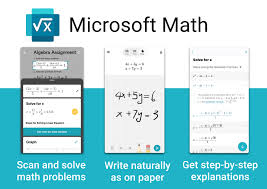# Microsoft Math Solver App for Students As A Learning Tool

Learn math with Microsoft Math Solver and free step-by-step instructions. Microsoft Math Solver instantly recognizes the problem and helps you to solve it with detailed step-by-step explanation, interactive graphs, similar problems from the web and online video lectures. it look up quickly related math concepts. It is FREEWARE, that is, absolutely FREE and NO ads.

Microsoft Math Solver App, also known as Microsoft Mathematics, is a freely downloadable educational program, designed for mobile platforms (iOS and Android), that allows users to solve math and science problems. Developed and maintained by Microsoft, it is primarily targeted at students as a learning tool.

Microsoft Math was originally released as a bundled part of Microsoft Student. It was then available as a standalone paid version starting with version 3.0. For version 4.0, it was released as a free downloadable product and was called Microsoft Mathematics 4.0. While no longer in active development or support, Microsoft Mathematics 4.0 can still be downloaded from the official Microsoft Website.

#### Microsoft Math Solver Features

• Write a math equation on screen as you naturally do on paper

● Scan printed or handwritten math photo

● Type and edit using advanced scientific math calculator

● Get interactive Step-by-Step explanation & Graphing calculator

● Import images with math equations from gallery

● Scan and Solve Math Worksheets with multiple problems

● Search the web for similar problems and video lectures

● Try math word problems

● Pre-Algebra: radicals and exponents, fractions, matrices, determinants

● Algebra: quadratic equations, system of equations, inequalities, rational expressions, linear, quadratic and exponential graphs

● Word problems on math concepts, number theory, probability, volume, surface area

● Basic Calculus: Summations, Limits, derivatives, integrals

● Statistics: Mean, Median, Mode, Standard Deviation, permutations, combinations

This students learning tool is very useful, especially for Nigeria candidates preparing for JAMB and WAEC examinations when practicing Past Questions

Tutorials on how to use the Microsoft Math Solver on different Math subjects such as:

• Algebra
• Trigonometry
• Statistics
• Matrices
• Calculus and many more is coming soon.

Study Past Question and Answers for UTME & SSCE

4 1 vote
Article Rating
Subscribe
Notify of